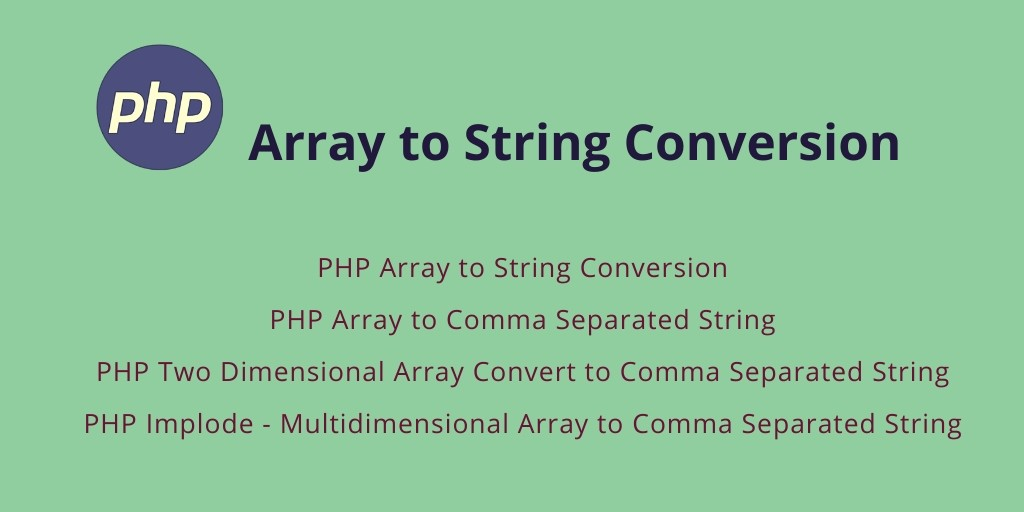Php 23-Jul-2021

### PHP array to comma-separated string

Here you will learn, php array to comma separated string, PHP Array to String Conversion, PHP Array to Comma Separated String, PHP Two Dimensional Array to String Conversion, PHP Implode – Multidimensional Array to Comma Separated String, PHP Implode Multidimensional Array to Comma Separated String.

This tutorial demonstrates, how to convert array to string in PHP using the PHP implode function.

## Array to String Conversion in PHP

In PHP, The implode function is used to convert an array into a string.

This tutorial shows you how to convert string to an array, two-dimensional array, and multi-dimensional in PHP.

### Syntax of using the PHP implode method

The basic syntax is of the implode function is:

` implode(separator,array)`

Parameters of implode function:

Parameter Description
separator Optional. Specifies what to put between the array elements. Default is “” (an empty string)
array Required. The array to join to a string

### Examples – Convert an Array to String in PHP

• PHP Array to String Conversion
• PHP Array to Comma Separated String
• PHP Two Dimensional Array Convert to Comma Separated String
• PHP Implode – Multidimensional Array to Comma Separated String

#### PHP Array to String Conversion

Let’s take an example for index array convert to string in PHP:

<?php

\$array = array("PHP","PYTHON","JAVA");

\$string =implode(" ", \$array);

echo "The returned string after implod: <br><strong>".\$string ."</strong>";

?>

##### Index array convert to String example source code

<!DOCTYPE html>
<html>
<title>PHP Array to String Conversion</title>
<body>
<?php

\$array = array("PHP","PYTHON","JAVA");

\$string =implode(" ", \$array);

echo "The returned string after implode with comma: <br><strong>".\$string ."</strong>";

?>

</body>
</html>

#### PHP Array to Comma Separated String

Let’s take new example with an array, here we will convert array to a comma-separated string.

<?php

\$array = array("PHP","PYTHON","JAVA");

\$string =implode(",", \$array);

echo "The returned string after implode with comma: <br><strong>".\$string ."</strong>";

?>

##### Array to Comma Separated String Example Source Code

<!DOCTYPE html>
<html>
<title>PHP Array to Comma Separated String</title>
<body>

<?php

\$array = array("PHP","PYTHON","JAVA");

\$string =implode(",", \$array);

echo "The returned string after implode with comma: <br><strong>".\$string ."</strong>";

?>

</body>
</html>

#### PHP Two Dimensional Array Convert to Comma Separated String

Now we will take the example of a two-dimensional array. In this example, we will convert two-dimensional array to comma separate string.

<?php
// Two Dimensional array
\$array = array (
array ('01','03','02','15'),
array ('05','04','06','10'),
array ('07','09','08','11'),
array ('12','14','13','16')
);

\$tmpArr = array();
foreach (\$array as \$sub) {
\$tmpArr[] = implode(',', \$sub);
}
\$result = implode(',', \$tmpArr);

echo \$result;

##### Two Dimensional Array to Comma Separated String Example Source Code

<!DOCTYPE html>
<html>
<title>PHP Two Dimensional Array to Comma Separated String</title>
<body>

<?php
// Two Dimensional array
\$array = array (
array ('01','03','02','15'),
array ('05','04','06','10'),
array ('07','09','08','11'),
array ('12','14','13','16')
);

\$tmpArr = array();
foreach (\$array as \$sub) {
\$tmpArr[] = implode(',', \$sub);
}
\$result = implode(',', \$tmpArr);

echo \$result;
?>

</body>
</html>

#### PHP Implode – Multidimensional Array to Comma Separated String

Here you will learn how you can convert multidimensional array to comma separated string.

<?php

\$multi_dimensional_array = array(

array(

'Name' => 'PHP',

'Email' => 'php@gmail.com'

),

array(

'Name' => 'Java',

'Email' => 'java@gmail.com'

)

);

echo implode(', ', array_map(function (\$string) {

return \$string['Name'];

}, \$multi_dimensional_array));

?>

##### Multi-Dimensional Array to Comma Separated String Example Source Code

<!DOCTYPE html>
<html>
<title>PHP Multi Dimensional Array Convert to Comma Separated String</title>
<body>
<?php

\$multi_dimensional_array = array(

array(

'Name' => 'PHP',

'Email' => 'php@gmail.com'

),

array(

'Name' => 'Java',

'Email' => 'java@gmail.com'

)

);

echo implode(', ', array_map(function (\$string) {

return \$string['Name'];

}, \$multi_dimensional_array));

?>

</body>
</html>

### Conclusion

Array to string conversion in the PHP tutorial. You have learned how to convert index, two dimensional, and multidimensional array to string or comma-separated string with example.